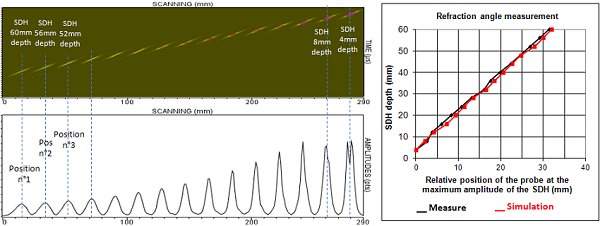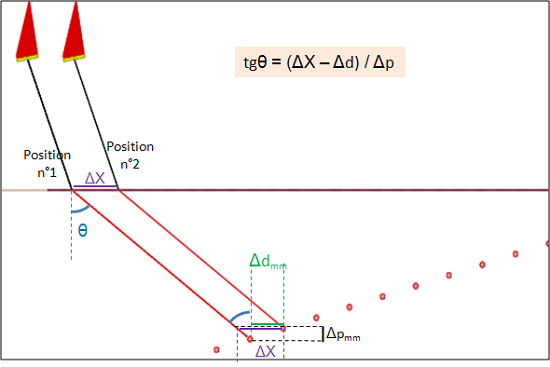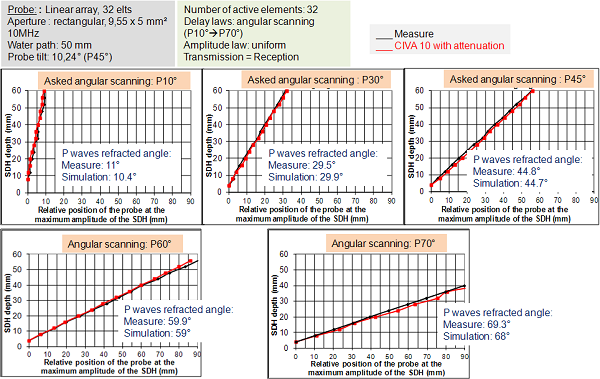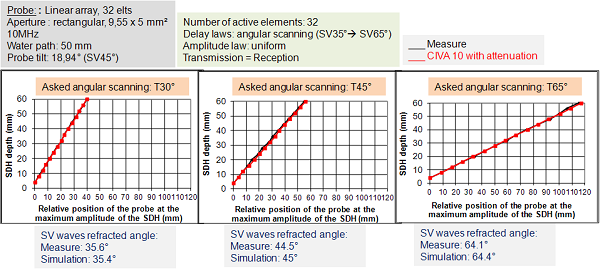UT - Phased Arrays - Results obtained with the sectorial scanning algorithm

This algorithm was evaluated with the 10 MHz immersion probe. According to the wave type (P or SV), the probe is tilted with a specific angle: 10.5° in order to generate a P45 refraction, 18.9° in order to generate a SV45 refraction.

Results obtained with the 10 MHz probe tilte at 10.2° (P45° refraction)

Delay law : sectorial scanning, first deflection angle: 0°, last deflection angle: 80°, P waves calculation for delay law, 32 active elements.

The water path is 50 mm and the probe is tilted with a 10.24° angle.

direct P echoes amplitude

The P wave simulated amplitude curves for SDHs show good agreement with the experimental amplitude curves whatever the attenuation law exponent (2 or 4). However, there is a difference between simulation and experiments on the SV amplitude curve measured for P60° deflection. The difference is less important with the attenuation law in exponent 2 but still remains significant (up to 5 dB difference).

Ø2 mm SDH amplitude comparison (experiment/CIVA), P direct mode

These dicrepancies between experiments and results are due to the simulated SV beam. P60° refraction induces a SV28° mode.However, The simulation of the SV beam shows a splitting at the water/steel interface which is not observed experimentally. The SV beam splitting is due to limitations of the model. The strong variation of the transmission coefficient close to critical angle splits the SV incident beam. Some waves are not considered which reduces the amplitude of the SV simulated echo. (cf. here ).

P wave echodynamic curves comparison

Simulated echodynamic curves with the law exponent 2 agree well with the experimental curves.

P waves refraction angle

The “desired” refraction angles when calculating the delay law will be compared to the “real” angles, that is to say those obtained after application of the law.

The values of these “real” refraction angles will be calculated from the (experimental or simulated) echodynamic curves obtained with SDHs:

The probe positions for which the SDHs amplitudes are highest are recorded (cf. figure below, bottom left).Exemplary curve of the position sensor for which the amplitude of the echo of a SDH at a given depth “p” is maximum according to “p”

Experimental and simulated curves on the previous figure (on the right) allow to appreciate the evolution of the refracted angle as a function of depth and allow to measure it.

The comparison of these two curves indicates if the refracted beam angle in the specimen is the same as the one predicted by CIVA.

The measurement of the “real” angle of the beam is done using the relationship described in the figure below.Real refracted beam angle in the specimen

The following figure compares the curves displaying the positions of the probe where the amplitude is maximal as a function of the SDH depth. Simulated curves agree well with the experimental one and experimental refraction angles (i.e. “real” angles) are in good agreement with the refraction angles requested in simulation (which are independent of the attenuation law).Experiment / CIVA comparison of refraction angles defined for delay law calculation from experiment or simulation (P echoes from SDH)

Results obtained with the 10 MHZ probe tilted at 18.9° (SV45° refraction)

Delay law: sectorial scanning, first deflection angle: 35°, last deflection angle: 65°, SV waves calculation for delay law, 32 active elements.

The water path is 50 mm.

Direct SV echoes amplitude

As it can be seen on the following figure, with SV waves, the attenuation law in exponent 2 provides amplitude curves close to the experimental results. However, whatever the exponent value, simulated SV echoes amplitudes differ from the experimental amplitudes. With the attenuation law in exponent 2, simulated amplitudes decrease faster than the experimental one and the deeper is the SDH, the larger is the difference (up to 6 dB).

SV waves A-scans and echodynamic curves Comparison

The two following figures compare the experimental and simulated Ascans and echodynamic curves obtained with the attenuation law in exponent 2. There is always a good agreement between simulation and experiments with this exponent.

Ø2 mm SDH Ascans comparison (experiment/CIVA), SV direct mode

Ø2 mm SDH echodynamic curves comparison (experiment/CIVA), SV direct mode

SV waves refraction angle

The refraction angle is measured according to the process described previously for P waves. The results obtained in SV mode are presented on the following figure:Experiment / CIVA comparison of refraction angles defined for delay law calculation from experiment or simulation (SV echoes from SDH)

There is a very good agreement between experimental and simulated refraction angles.

CONCLUSION

Comparisons simulation / experiment show that there are differences with the 10 MHz probe when it is used for SV angular scanning. These differences (especially on the amplitude evolution) are reduced but do not disappear when using the attenuation law in exponent 2.

However, whatever the wave type (P or SV), the experimental (“real”) refraction angles are in good agreement with the one desired and which are independant of the chosen attenuation law. The delay laws provided by CIVA in order to deviate the acoustical beam of a phased array allow to obtain de desired deviation.

Back to Phased arrays